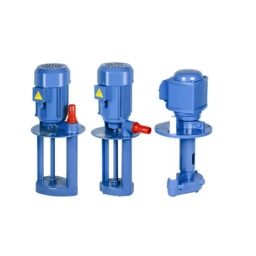# Simultaneously 81441

The tank is filled through three openings, A, B, and C. The simultaneously open inlets A and B are filled in 1 hour, inlets A and C in 45 minutes, and inlets B and C in 1.5 hours. How long would it take to fill each inlet separately?

a =  1.2 h
b =  6 h
c =  2 h

### Step-by-step explanation:Did you find an error or inaccuracy? Feel free to write us. Thank you!

Tips for related online calculators
Looking for calculator of harmonic mean?
Looking for a statistical calculator?
Do you have a linear equation or system of equations and looking for its solution? Or do you have a quadratic equation?
Do you want to convert time units like minutes to seconds?